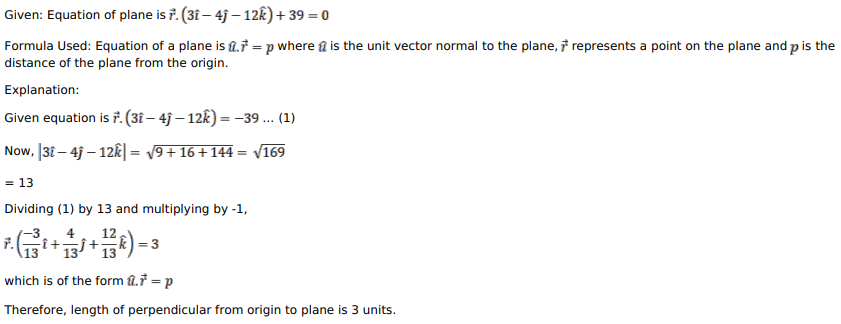# Mark against the correct answer in each of the following:

Question:

Mark against the correct answer in each of the following:

The length of perpendicular from the origin to the plane $\overrightarrow{\mathrm{r}} \cdot(3 \hat{\mathrm{i}}-4 \hat{\mathrm{j}}-12 \hat{\mathrm{k}})+39=0$ is

A. 3 units

B. $\frac{13}{5}$ units

C. $\frac{5}{3}$ units

D. None of these

Solution: